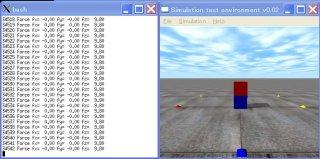# ODE Tutorial 13: Let's make a pressure sensor !It continues to the last time, utilizes the dJointFeedback structure. This time the joint (the joint) the sample cord/code which acquires the power which catches is introduced.

When this is applied, you attach to the sole of a humanoid robot, you can simulate the pressure sensor which is utilized in the measurement of ZMP.

• void dJointSetFeedback (dJointID and dJointFeedback *);
The dJointFeedback structure is set to the joint JointID which acquires the information of power and torque.
typedef struct dJointFeedback {
dVector3 f1;    // The power which the joint has caused to body 1
dVector3 t1;    // The torque which the joint has caused to body 1
dVector3 f2;    //  The power which the joint has caused to body 2
dVector3 t2;    // The torque which the joint has caused to body 2
} dJointFeedback;
• dJointFeedback *dJointGetFeedback (dJointID);
Information of power and torque of the joint which it is designated with dJointID is acquired.

Next, the sample program which used this API is introduced. It is the program which indicates the power which the fixed joint (Fixed Joint) with attaches two boxes, depends on there. It meaning that weight of the box is each 1kg, z axial direction (upper part direction) the power of 9.8N being applied, you insert and it is according to theory. With my environment according to theory it had started hitting the value of 9.8.

Furthermore, in the program you regard among and under two boxes the pressure sensor. When the pressure sensor of the humanoid robot”s  sole is simulated, size of the box under the paragraph and number should have been increased small reason are.

[code]
// sensor.cpp by Kosei Demura 2006-7-26
#include
#include

static dWorldID world;
static dSpaceID space;
static dGeomID ground;
static dJointID fixed;
static dJointGroupID contactgroup;
dJointFeedback *feedback = new dJointFeedback;
dsFunctions fn;

typedef struct {
dBodyID body;
dGeomID geom;

static void nearCallback (void *data, dGeomID o1, dGeomID o2)
{
static int MAX_CONTACTS = 10;
int i;

// do not collide if two bodies are conneted by a joint
dBodyID b1 = dGeomGetBody(o1);
dBodyID b2 = dGeomGetBody(o2);
if (b1 && b2 && dAreConnected (b1,b2)) return;

dContact contact[MAX_CONTACTS]; //
int numc = dCollide(o1,o2,MAX_CONTACTS,&contact.geom,
sizeof(dContact));
if (numc > 0) {
for (i=0; i
contact[i].surface.mode = dContactSoftCFM | dContactSoftERP;
contact[i].surface.mu = dInfinity;　　//
contact[i].surface.soft_cfm = 1e-8;
contact[i].surface.soft_erp = 1.0;
dJointID c = dJointCreateContact(world,contactgroup,&contact[i]);
dJointAttach (c,dGeomGetBody(contact[i].geom.g1),
dGeomGetBody(contact[i].geom.g2));
}
}
}

static void simLoop (int pause)
{
static int steps = 0;

dSpaceCollide(space,0,&nearCallback);
dWorldStep(world,0.01);
dJointGroupEmpty(contactgroup);

feedback = dJointGetFeedback(fixed);　// get force and torque information
printf(“%5d Force fx=%6.2f “,steps++,feedback->f1);　// x coordinate
printf(“fy=%6.2f “,feedback->f1);　　　　　　　　　　　　　// y coordinate
printf(“fz=%6.2f \n”,feedback->f1);　　　　　　　　　　　　// z coordinate

// draw a box
dsSetColor(1.0,0.0,0.0);
dReal sides1[] = {box.length,box.width,box.height};
dsDrawBoxD(dBodyGetPosition(box.body),
dBodyGetRotation(box.body),sides1);

// draw a sensor
dsSetColor(0.0,0.0,1.0);
dReal sides2[] = {sensor.length,sensor.width,sensor.height};
dsDrawBoxD(dBodyGetPosition(sensor.body),
dBodyGetRotation(sensor.body),sides2);
}

void start()
{
static float xyz = {0.0,-3.0,1.0};
static float hpr = {90.0,0.0,0.0};
dsSetViewpoint (xyz,hpr);
}

void setDrawStuff() {
fn.version = DS_VERSION;
fn.start = &start;
fn.step = &simLoop;
fn.command = NULL;
fn.stop = NULL;
fn.path_to_textures = “../../drawstuff/textures”;
}

int main (int argc, char **argv)
{
setDrawStuff();

world = dWorldCreate();
space = dHashSpaceCreate(0);
contactgroup = dJointGroupCreate(0);
dWorldSetGravity(world,0,0,-9.8);

ground = dCreatePlane(space,0,0,1,0);

dMass m1;
dReal x0 = 0.0, y0 = 0.0, z0 = 0.0;

// a sensor (blue box)
sensor.length = 0.2; sensor.width = 0.2;
sensor.height = 0.2; sensor.mass = 1.0;
sensor.body = dBodyCreate(world);
dMassSetZero(&m1);
dMassSetBoxTotal(&m1,sensor.mass,sensor.length,sensor.width,sensor.height);
dBodySetMass(sensor.body,&m1);
dBodySetPosition(sensor.body, x0, y0, 0.5 * sensor.height + z0);

sensor.geom = dCreateBox(space,sensor.length,sensor.width,sensor.height);
dGeomSetBody(sensor.geom,sensor.body);

// a box (red box）
box.length = 0.2; box.width = 0.2;
box.height = 0.2; box.mass = 1.0;
box.body = dBodyCreate(world);
dMassSetZero(&m1);
dMassSetBoxTotal(&m1,box.mass,box.length,box.width,box.height);
dBodySetMass(box.body,&m1);
dBodySetPosition(box.body, x0, y0, sensor.height + 0.5 * box.height + z0);

box.geom = dCreateBox(space,box.length,box.width,box.height);
dGeomSetBody(box.geom,box.body);

// a fixed joint
fixed = dJointCreateFixed(world,0);
dJointAttach(fixed,box.body,sensor.body);
dJointSetFixed(fixed);

// Set a joint to retrieve force and torque information
dJointSetFeedback(fixed,feedback);

dsSimulationLoop(argc,argv,352,288,&fn);
dWorldDestroy(world);
return 0;
}

[/code]

english
スポンサーリンク
demura.netをフォローするdemura.net

### Folding@home Kanazawa (ID 257261)

みんなのためにおうちで新型コロナウイルスを解析しよう！

タイトルとURLをコピーしました